Examples

Chapter 10 Class 7 Practical Geometry
Serial order wise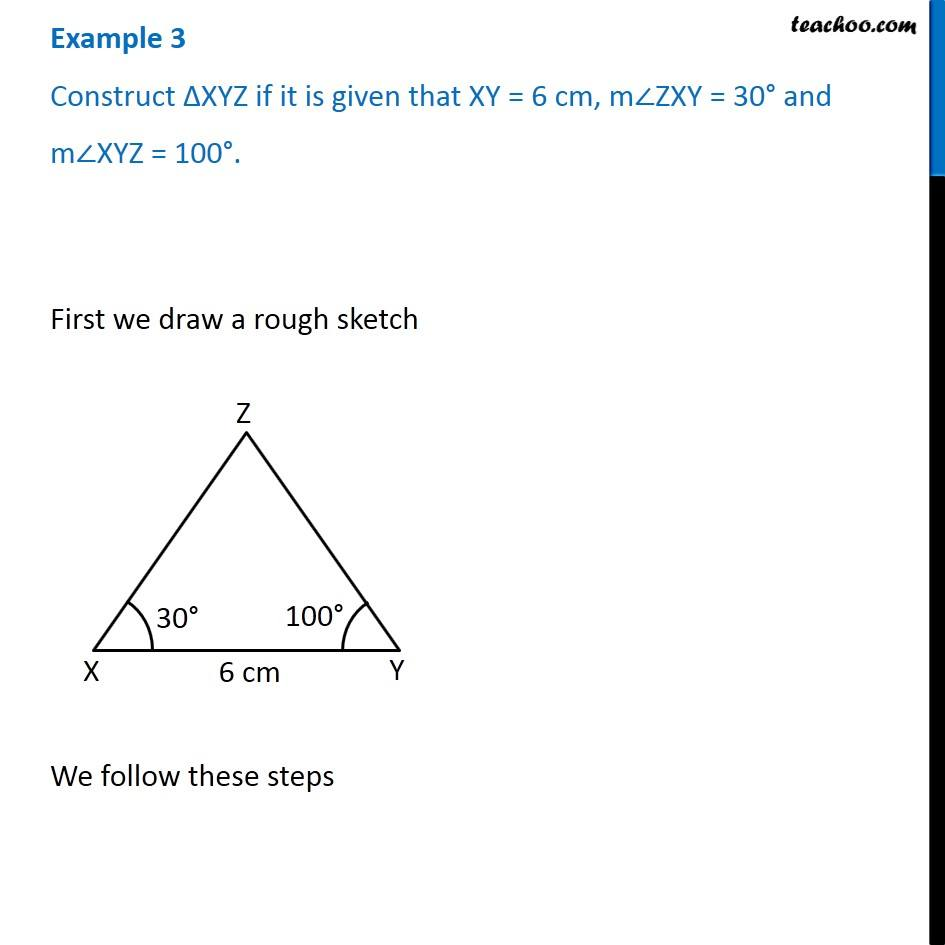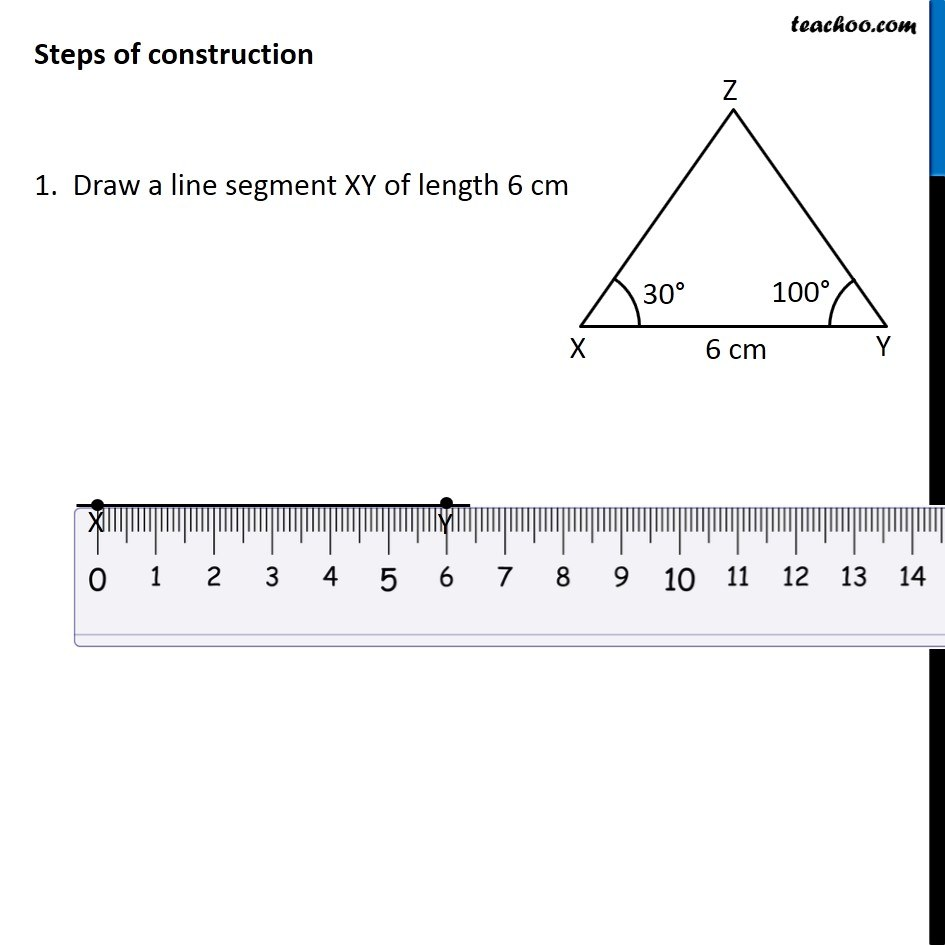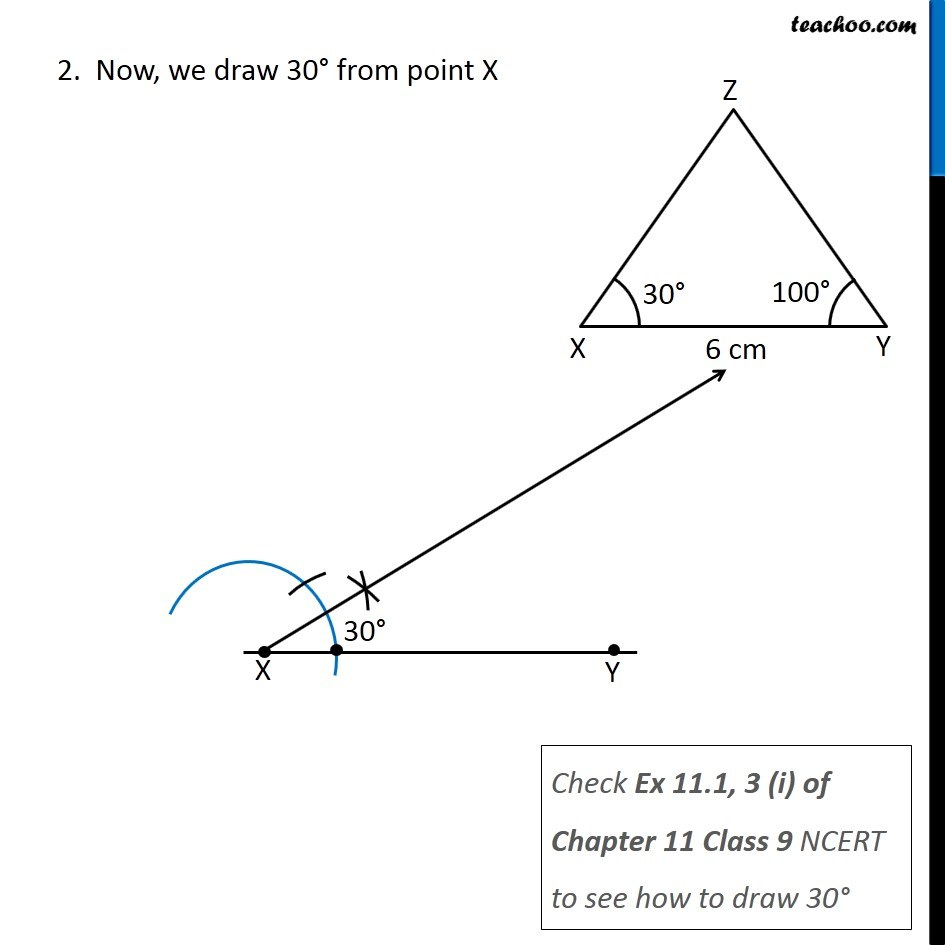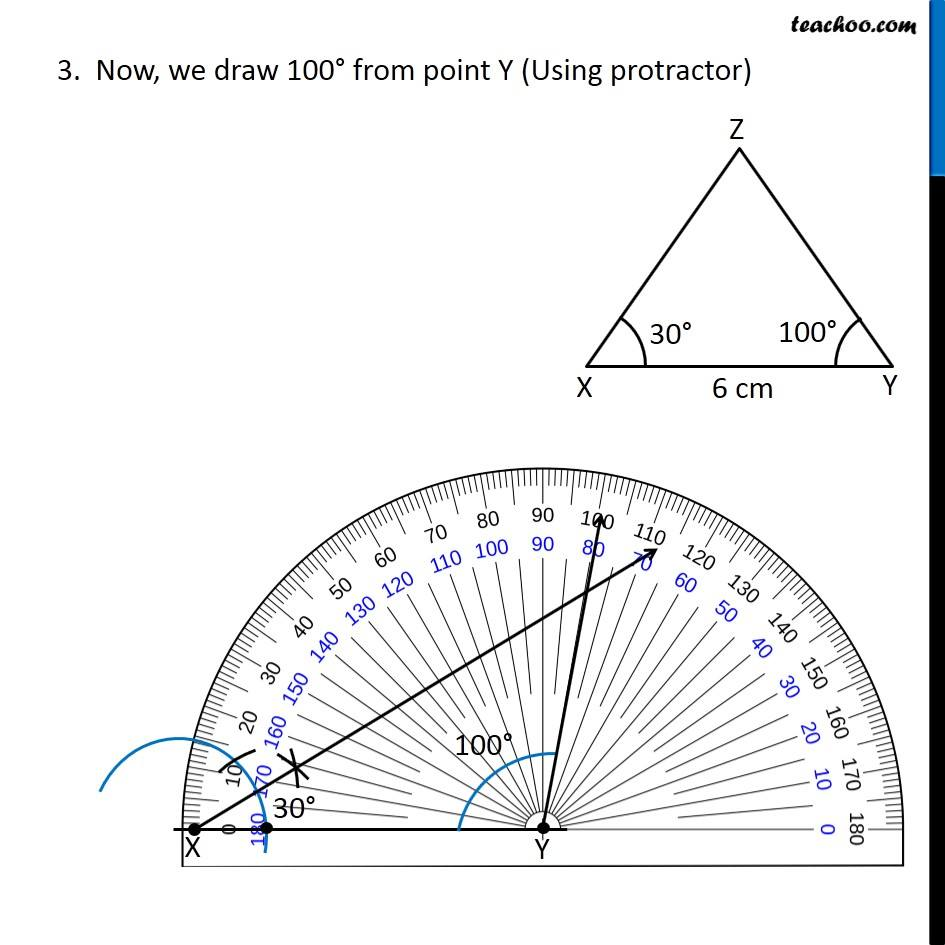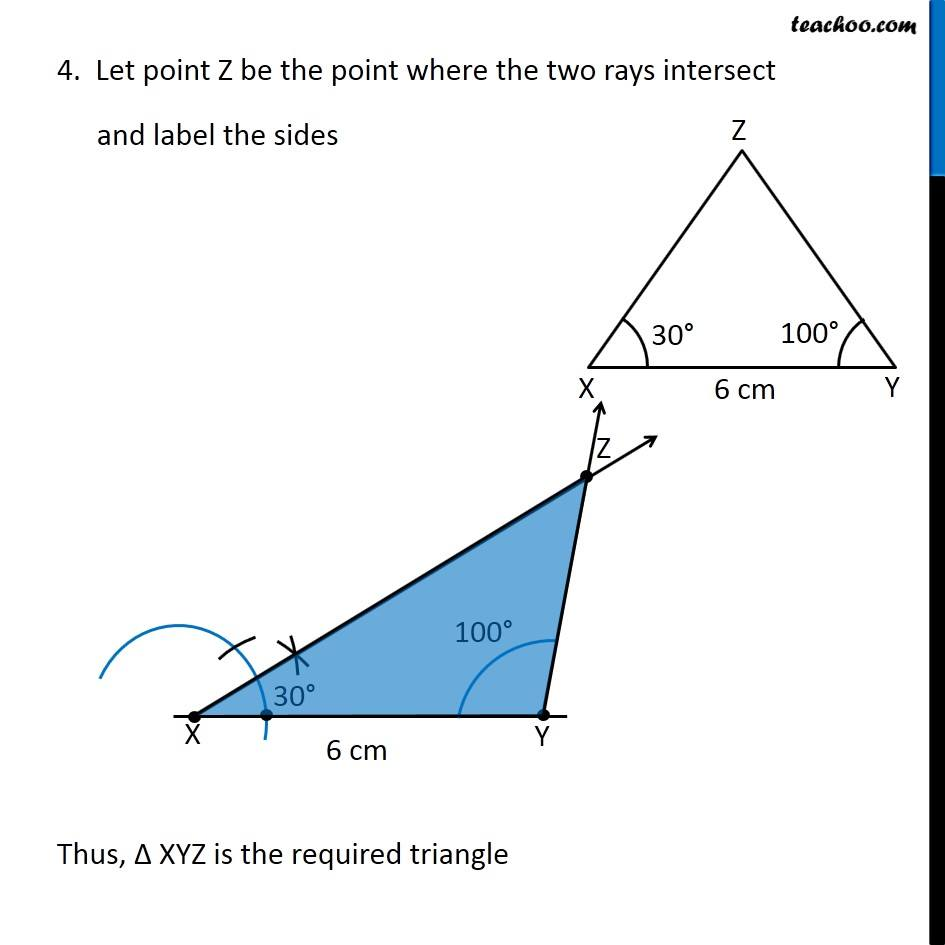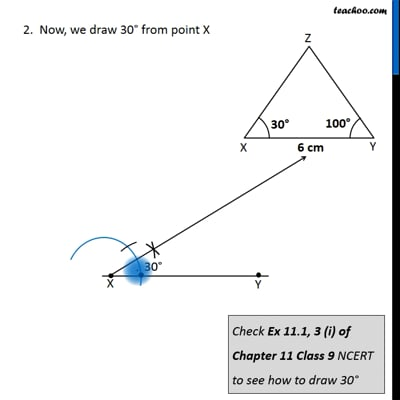This video is only available for Teachoo black users

Get live Maths 1-on-1 Classs - Class 6 to 12

### Transcript

Example 3 Construct ∆XYZ if it is given that XY = 6 cm, m∠ZXY = 30° and m∠XYZ = 100°. First we draw a rough sketch We follow these steps Steps of construction 1. Draw a line segment XY of length 6 cm 2. Now, we draw 30° from point X Check Ex 11.1, 3 (i) of Chapter 11 Class 9 NCERT to see how to draw 30° 3. Now, we draw 100° from point Y (Using protractor) 4. Let point Z be the point where the two rays intersect and label the sides Thus, Δ XYZ is the required triangle